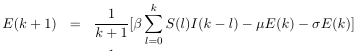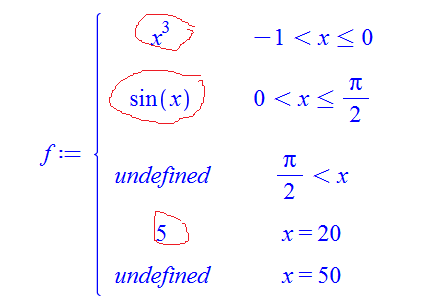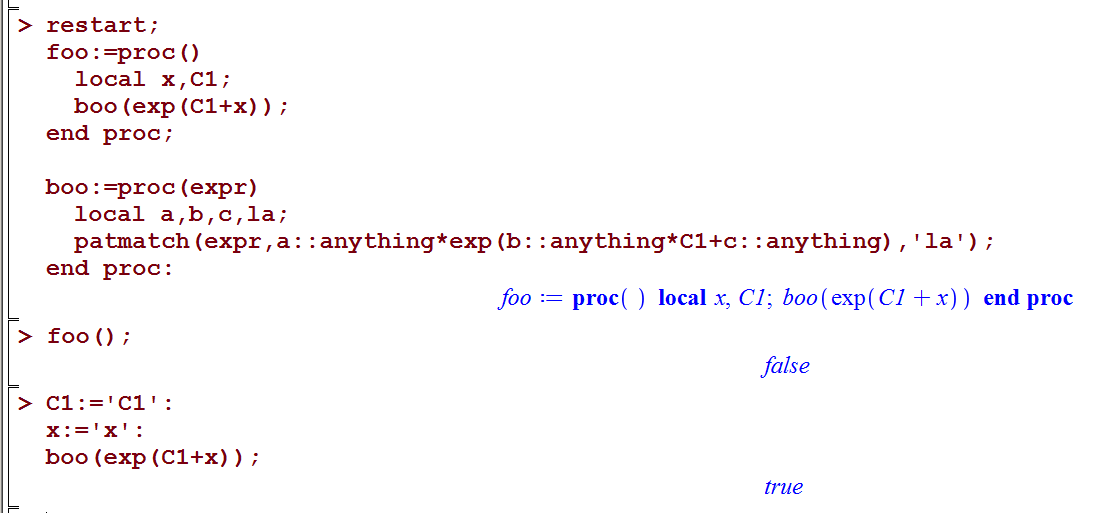## how to use .hdb Maple help file. Trying to find he...

http://algo.inria.fr/libraries/obsolete-releases.html

Downloaded the .mla and the .hdb files. Put them in current directory where my worksheet .mw is.

Opened the .mw and typed

restart;
libname := currentdir(), libname;
_algolibcontent();

Content of algolib (version 14.0), as of October 2010:

+ encyclopedia.            [Written by Stéphanie Petit, with contributions by Bruno Salvy and Michèle Soria.]
+ gdev.                [Written by Bruno Salvy.]
+ gfun (version 3.53).        [Maintained and extended by Bruno Salvy, with contributions by Ludovic Meunier, Marc Mezzarobba, Marni Mishna, and Eithne Murray, original version by Bruno Salvy and Paul Zimmermann.]
+ Holonomy (version 3.4).    [Written by Frédéric Chyzak.]
+ MAD (version 1.445).        [Written by Ludovic Meunier.]
+ Mgfun (version 4.1).        [Written by Frédéric Chyzak, with contributions by Shaoshi Chen, Cyril Germa, Lucien Pech, and Ziming Li.]
+ MultiSeries.            [Written by Bruno Salvy.]
+ regexpcount (version 1.5).    [Written by Pierre Nicodème.]

Ok. Now how to obtain help? I can call one of its functions, like this

\frac{1}{2}

But I do not know how to find help on MADLaTeX:-latex since ?MADLaTeX does nothing and ?MADLaTeX:-latex does nothing.

How to obtain the help pages for this package? I looked online and do not see anything. I do not know what to do with the .hdb file that I downloaded. I am using Maple 2018 and it does not seem to support .hdb files anyway.

Does I need to go through all the conversion steps described in

https://www.maplesoft.com/support/help/Maple/view.aspx?path=HelpTools%2fMigrate

just to see help on one function?

I just need to find how to call is Latex function and if it has any options. Any one knows an online page that have these on it?

## How can I check six numbers are length six sides o...

How can I check six numbers: a, b, c, d, e, f are length six sides of a tetrahedron?

## How to find the sum of prime factors?...

I am working with sequences and sometimes need to calculate the sum of prime factors (with multiplicity) of a number n. Example, n=12, answer = 2+2+3 = 7.

Any suggestions?

thanks

David.

## Plotting differential equations...

i need to plot this equation but can't find a way to do so. Can anyone help?beta parameter and initial value of S(k), E(k) is known, but i have problems at the sum and (k+1)..

## Animating multiple variables at once...

Hello!

I would like animate a function with multiple variables. At the moment my code in maple is:

plots[animate]( plot, [A*sin(B*x), x=0..10], A=0..2, B=0..2 );

I would like it to increase in amplitude when I run the A animation, and increase in frequency when I run the B animation. I want them to both be in the same graph , say have A=0.5 while B=0.5. then I could run the B animation with the amplitude fixed.

It would also be great to run them both at the same time.

Can someone help me?

-Thanks!!!

P.S: I'd eventually like to manipulate "implicitplot3d" with multple variables being animated at the same time.

hallo everyone, I'm working on a project for the Uni, and I'm facing the flowing problem.

I have Test-Matrix [3*12] called Testna it contains random nummbers( random because i wantend test the loop, the real matrix is much complexer ).

Testna := RandomMatrix(3, 12)

and I have a Vektor called Z

> Z:=Vector[row](5, {(1) = z, (2) = z-2, (3) = z-4, (4) = z-6, (5) = z-8})

>k:=4

Z and K are paramter previously in the worksheet

and I want to create a Matrix CC[3*3] using a nested loop so that the cofficient of CC(i,j) are as following

so for i =1 , j=1 and n from 1 to k=4  I wanted to have

CC(1,1):= Testna(1,1)*(Z(1,1)-Z(1,2))  +  Testna(1,4)*(Z(1,2)-Z(1,3) +Testna(1,7)*(Z(1,3)-Z(1,4))  +  Testna(1,10)*(Z(1,4)-Z(1,5)

so for i =1 , j=2 and n from 1 to k=4  I wanted to have

CC(1,2):= Testna(1,2)*(Z(1,1)-Z(1,2))  +  Testna(1,5)*(Z(1,2)-Z(1,3) +Testna(1,8)*(Z(1,3)-Z(1,4))  +  Testna(1,11)*(Z(1,4)-Z(1,5)

so for i =1 , j=3 and n from 1 to k=4  I wanted to have

CC(1,3):= Testna(1,3)*(Z(1,1)-Z(1,2))  +  Testna(1,6)*(Z(1,2)-Z(1,3) +Testna(1,9)*(Z(1,3)-Z(1,4))  +  Testna(1,12)*(Z(1,4)-Z(1,5)

so for i =2 , j=1 and n from 1 to k=4  I wanted to have

CC(2,1):= Testna(2,1)*(Z(1,1)-Z(1,2))  +  Testna(2,4)*(Z(1,2)-Z(1,3) +Testna(2,7)*(Z(1,3)-Z(1,4))  +  Testna(2,10)*(Z(1,4)-Z(1,5)

so for i =2 , j=2 and n from 1 to k=4  I wanted to have

CC(2,2):= Testna(2,2)*(Z(1,1)-Z(1,2))  +  Testna(2,5)*(Z(1,2)-Z(1,3) +Testna(2,8)*(Z(1,3)-Z(1,4))  +  Testna(2,11)*(Z(1,4)-Z(1,5)

so for i =2 , j=2 and n from 1 to k=4  I wanted to have

CC(2,3):= Testna(2,3)*(Z(1,1)-Z(1,2))  +  Testna(2,6)*(Z(1,2)-Z(1,3) +Testna(2,9)*(Z(1,3)-Z(1,4))  +  Testna(2,12)*(Z(1,4)-Z(1,5)

and so on.. for i=3, j=3 and n from 1 to K=4

the Problem is that the loop doesnt work as I wanted(above)  and I dont know why, so insted of having a Matrix CC:=[  [C(1,1), C(1,2) C(1,3),], [C(2,1), C(2,2) C(2,3),], [C(3,1), C(3,2) C(3,3)]  ] I become a Matrix CC:=[ [C(1,1), C(1,1) C(1,1),],[C(2,2), C(2,2) C(2,2),],[C(3,3), C(3,3) C(3,3),]]

here is the Maple code

Test := proc ()
local i, j, n; global CC, Testna, Z, k:
CC := Matrix(3, 3);
for i from 1to 3 do for
j from 1 to 3 do
for n from 1 to k do
CC(i, j) := CC(i, j)+Testna(i, 1+(n-1)*3)*(Z(1, n)-Z(1, n+1))
end do
end do
end do
end proc():
CC

## how to filter out undefined result from piecewise ...

After solving something, sometimes the result comes in piecwise. I'd like to just obtain all those results which are not undefined, into a list, so I can process them more easily. I am not sure what is the correct way to do this in Maple.

Here is an example.

restart;
f := piecewise(x>-1 and x<=0, x^3,
x>0 and x<=Pi/2, sin(x),
x>Pi/2, undefined,
x=20, 5,
x=50, undefined);I need to pick all entries that do not have "undefined". I do not need the rhs condition  which is x<=1. So for the above, I'd like to obtain

sol:=[ x^3, sin(x), 5]

If there more entries which are also not undefined, they go into the list in order.

when I do

sol:=convert(sol,pwlist,x);

This gives

sol := [0,-1,x^3,0,sin(x), (1/2)*Pi,undefined,20,undefined,20,undefined,50,undefined,50,undefined]


Now it gets confusing. How to change the above to

sol:=[ x^3, sin(x), 5]

I do not even understand the conversion result above. I do not know why it gives 50 twice. And I do not even see the "5" in there. So I do not know how to decode this now.

Update:

I found this solution. But it might not be best:

contents :=[op(f)];
[seq(if(contents[i]=undefined,NULL,contents[i]),i=2..nops(contents),2)];


gives

[x^3, sin(x), 5]

## Suppress dependency of a function while exporting ...

Dear all,

UsingI can suppress the dependency ofin the differential equationin the output when I typeHowever, when I want to export the output to LaTeX or MathML usingand, the dependency onis still there!

How to remove the dependency on?

Thank you!

## inert form of operator...

When I have an Operator like

eq:=1/r*diff(r*diff(f(r),r),r)

typically maple evaluates/expands it by product rule.

How can I tell him to not do it?

I tried using the inert operator % but probably i didn't do it right.

## How can I do some statistics on the indicators ret...

Hi everybody,

I want to compare the performances of a set of codes that realize the same operation.
The worksheet I use is organized this way

restart:
Code1 := proc(...) ... end proc:
CodeTools[Usage](Code1(...))

restart:
Code2 := proc(...) ... end proc:
CodeTools[Usage](Code2(...))

and so on.

It appears that the execution of the whole worksheet doesn't always return the same values for the different indicators, which means that the ranking of the codes can be affected

To obtain more precise indicators I thought do do some statistics.
I then replace each bloc by :

restart:
CodeX := proc(...) ... end proc:  # here X stands for 1, 2, ...
N := "some positive integer"
for n from 1 to N do
CodeTools[Usage](CodeX(...))
"catch the values of the indicators"
end do:
"compute some statistics on the samples ot these indicator"

The problem is that Usage doesn't return any values but only does a printf.
I investigated two ways to circumvent this problem:

1. - redirect the printings to file MyFile by using writeto(MyFile)
- extract the numerical values of the indicators
- compute the desired statistics
It works well but it is far from being elegant

2. - insert with(CodeTools) after the restart (within a separate block)
- copy-paste the output of showstat(Usage) to define a new function, let's say MyUsage
- insert at the correct place some lines like
Tcpu := CodeTools:-FormatTime(result:-cputime)  (if my time of interest is the cpu time)
(this for all the indicators)
- insert the line  [..., Tcpu, ...]; before the 'end proc' declaration;
More elegant but it doesn't work
I get the error   error (in T) DoUsage is not a command of the CodeTools package

Do you have some idea (beyond the first method above) of the way to catch the performance indicators that Usage computes ?

## Pick the "positive" solution of a quadratic...

Quadratic equation ax^2 + bx + c = 0. Coeff's long and ugly expressions in their own right, but I know that a is nonzero, that there is at least one real solution, and that I want the largest.

So, question: How do I get Maple to return the -b/2a PLUS sqrt((b/2a)^2-c/a) solution?

## patmatch fails when called from another proc() but...

I am learning patmatch. And found strange problem.

When I make an expression inside a proc A, and pass this expression to another proc B, which then uses patmatch  on it, the pattern fails to match.

but calling proc B directly, with exactly same expression, patmatch does match.

This is confusing and not sure why it happens. It seems related to using symbol which was declared local vs. same symbol but is global. Here is an example

restart;
foo:=proc()
local x,C1;
boo(exp(C1+x));
end proc;

boo:=proc(expr)
local a,b,c,la;
patmatch(expr,a::anything*exp(b::anything*C1+c::anything),'la');
end proc;


Call to foo() returns false. But call to boo() returns true. Even though I am using same expression.

foo();
false

C1:='C1':
x:='x':
boo(exp(C1+x));
trueWhat Am I doing wrong, and how to make it work in both cases?

## Renaming constants...

Not sure if it's the right place to ask this very simple question.

I have solved a DE and got x(t) = _C1*sin(sqrt(k)*t/sqrt(m)). Both _C1 and sqrt(k/m) are constants but I want to use different letters for them. I want _C1 to be renamed A and sqrt(k/m) to be renamed W. Can someone please suggest how I can do this ?

Thanks.

## A quick way to make an interactive plot?...

Hello everyone!

I miss a convinient way to build interactive plots with parameters assigned to sliders. E.g. of such expressions as "a*sin(b*x+c)". Before Maple 2017 I could just right-click the expression, choose "plot builder" and then select "interactive plot with three parameters". That's it. Since the introduction of the new interactive plot builder I am at a loss because that way have been lost and I don't know any other way equally quick and convinient. "Interactive plot builder" now means quite another thing!

Any suggestions?